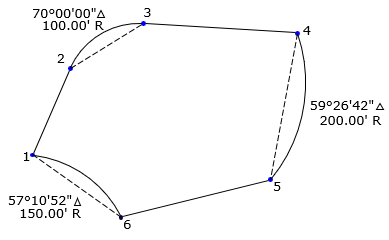### 2. Traverse with curves

#### a. Segments and sectors

Area by coordinates is constrained to following straight lines. Where curves are involved, Figure H-3(a), the method returns an area bounded by their chords, Figure H-3(b).(a) Traverse(b) Area by coordinates Figure H-3 Traverse with curves

To obtain the correct area, sector or segment areas are computed and added or subtracted accordingly. For the traverse in Figure H-3(a), the two segment areas are computed, Figure H-4(a),  and one subtracted from, the other added to, the area by coordinates, Figure H-4(b).(a) Segments(b) ATotal=A1-A2+A3 Figure H-4 Accounting for segments

#### b. Example area computation

Given the traverse in Figure H-5, determine its area.Figure H-5 Traverse with curves

In the table below, the points and their coordinates are in the first three columns; their cross-products are in the last two.

 Point North (ft) East (ft)1 500.00 1200.00 737,634 2 614.70 1249.70 624,850. 843,243 3 674.76 1347.43 828,261 892,620. 4 662.46 1552.76 1,047,730 725,778 5 467.41 1516.87 1,004,860 633,075 6 417.36 1317.39 615,766 658,696 1 500.00 1200.00 500,828 sums: 4,491,046 4,622,300.

The area isThis is the area bounded by the chords. We must account for the segments.

Recall that the area of a segment, bounded by an arc and its chord, isEquation H-2

Compute the area of each segment

Arc 2-3Arc 4-5Arc 6-1Total traverse area is 68,790 sq ft.

#### c. Segment or sector?

Consider the curvinlinear traverse in Figure H-5:Figure H-5 Curvilinear traverse

Its area can be computed using the arc sector, Figure H-6,(a) Area to radial lines, AR (b) Sector area, ASec Figure H-6 Area = AR + ASec

or using the arc segment, Figure H-7(a) Area to chord, AC (b) Segment area, ASeg Figure H-7 Area = AC + ASeg

Either method is fine. The only difference is that sectors require computing arc radius points, Figure H-6(a); segments do not, Figure H-7(a).